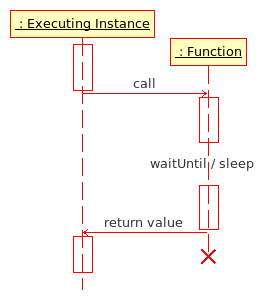# Difference between revisions of "Function"

A function is something defined in code which carries out an instruction or set of instructions and may or may not have inputs and outputs. The builtin functions which are provided by the various games themselves are referred to as commands, and are called in a different manor to functions which are defined within scripts. Functions were first introduced in the OFP: Resistance patch.

Functions can also be used to reuse code. You can write some code once in the function and then include it in many different scripts. When the code is updated, it is updated for all scripts. When you only copy and paste the code to the other scripts, you have to update every script on any change.

## Anatomy of a function

When scripting, there are two types of functions: functions-as-files and inline functions. Functions-as-files are instances where the a whole file itself is used to house a function, whereas inline functions are either contained within a variable or as a parameter of a function. Some built-in functions require functions-as-files, whereas most will support both.

### Parameters

Parameters for functions are available to the function via the magic variable _this. There is no implicit declaration of function parameters in scripting, nor variable typing. Common practice for defining parameters is done via the use of private variables and defined variables.

```//MyCode.sqf
private ["_parameterOne","_parameterTwo"];
```
```_parameterOne = _this select 0;
_parameterTwo = _this select 1;
```
```// Inline Function
MyInlineFunction ={
private ["_parameterOne","_parameterTwo"];

_parameterOne = _this select 0;
_parameterTwo = _this select 1;
};
```

### Return Values

The value of the last statement provided in a function is returned to the calling instance. In order for a return value to work correctly, there must not be a trailing semicolon after the statement.

```//MyCode.sqf
private ["_myName","_returnMe"];
_myName = _this select 0;

_returnMe = "FAIL";

if(_myName == "Test") then
{
_returnMe = "PASS";
};
_returnMe
```
```// myCodeInline
MyCodeReturnValue ={
private ["_myName","_returnMe"];
_myName = _this select 0;
_returnMe = "FAIL";

if(_myName == "Kaboom") then
{
_returnMe = "PASS";
};

_returnMe

};

_myCalledVariable = ["Kaboom"] call MyCodeReturnValue; // "PASS"
_myCalledVariableFail = ["Blah"] call MyCodeReturnValue; // "FAIL"
```
```//return.sqf
STATEMENT 1;
STATEMENT 2;
RETURN_VALUE
```
```//test.sqf
value = call compile preprocessFile "return.sqf";
// value is now RETURN_VALUE

call compile preprocessFile "return.sqf";
// valid, but RETURN_VALUE is not saved anywhere
```

### ExecutionFunction Execution Diagram in scheduled environment

Executing Instance : script, function or game engine

Functions can be executed from several points in the game:

Functions are first loaded as String from a file via preprocessFile or loadFile. They are then executed via the call or spawn command. Since Armed Assault the loaded String needs to be compiled in order to convert it to Code, which is required for call or spawn.

#### Call

Example (Operation Flashpoint):

```myFunction1 = loadFile "myFunction1.sqf";
myFunction2 = preprocessFile "myFunction2.sqf";

call myFunction1;
[1, 2] call myFunction2;
```

Example (Armed Assault):

```myFunction1 = compile loadFile "myFunction1.sqf";
myFunction2 = compile preprocessFile "myFunction2.sqf";

_result1 = call myFunction1;
_result2 = [1, 2] call myFunction2;
```

Functions executed using call are run within the executing instance, which waits for the result of the function. Unlike scripts, functions halt all other game engine processes until the function has completed its instructions. This means functions run faster than scripts, and the result of functions is immediate and unambiguous. It can also mean that if a function takes too long to run it will have an adverse effect on game play - large functions or CPU intensive functions can cause the game to seize up until it completes. When creating a functions you want the function to be short and sweet to achieve the best results.

Note: You can still use the special variables and commands of scripts in functions (Armed Assault only)!

#### Spawn

Functions may also be executed using spawn, but then the function result is not accessible, making it behave more like a procedure. Spawned functions will run asynchronously or alongside the executing instance. This helps prevent large CPU intensive functions from seizing up the game.

Example (Armed Assault):

```myFunction1 = compile loadFile "myFunction1.sqf";
myFunction2 = compile preprocessFile "myFunction2.sqf";

_param spawn myFunction1;
[1, 2] spawn myFunction2;
```

## Examples

### Example 1: max.sqf

In this example the function returns maximum of first and second argument.

max.sqf

```comment "Return maximum of first and second argument";
private ["_a","_b"];
_a = _this select 0;
_b = _this select 1;
if (_a>_b) then {_a} else {_b}
```

alternative max.sqf (big boys code :))

```(_this select 0) max (_this select 1)
```

executing script:

```fMax = compile preprocessFile "max.sqf";
maxValue = [3,5] call fMax;

// maxValue is now 5
```

### Example 2: infantrySafe.sqf

In this example the function returns no value and switches all units to safe mode.

```comment "Switch all infantry units to safe mode";
{
if (vehicle _x == _x) then
{
_x setBehaviour "safe"
}
} forEach _this
```

### Example 3: Inline Function

An inline-function can be created in any script:

```FNC_sayhello = {hint format["hello %1",_this]};
```

This function can then be called (in other scripts, functions, unit's init lines, trigger activation fields, etc.) via:

```name player call FNC_sayhello
```

In case the function doesn't require any arguments you can just call the function.

```call FNC_helloall
```